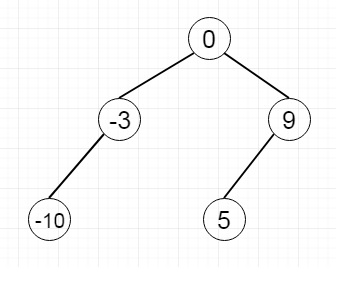# Convert Sorted Array to Binary Search Tree in Python

Suppose we have one sorted array A. We have to generate one height-balanced binary search. In this problem, a height-balanced binary tree is actually a binary tree in which the depth of the two subtrees of every node never differs by more than 1. Suppose the array is like [-10, -3, 0, 5, 9]. So one possible output will be like: [0, -3, 9, -10, null, 5]To solve this, we will follow these steps.

• If A is empty, then return Null
• find the mid element, and make it root
• Divide the array into two sub-arrays, left part of the mid element, and right part of the mid element
• recursively perform the same task for the left subarray and right subarray.

Let us see the following implementation to get a better understanding −

## Example

Live Demo

class TreeNode:
def __init__(self, data, left = None, right = None):
self.data = data
self.left = left
self.right = right
def insert(temp,data):
que = []
que.append(temp)
while (len(que)):
temp = que
que.pop(0)
if (not temp.left):
if data is not None:
temp.left = TreeNode(data)
else:
temp.left = TreeNode(0)
break
else:
que.append(temp.left)
if (not temp.right):
if data is not None:
temp.right = TreeNode(data)
else:
temp.right = TreeNode(0)
break
else:
que.append(temp.right)
def make_tree(elements):
Tree = TreeNode(elements)
for element in elements[1:]:
insert(Tree, element)
return Tree
def height(root):
if root is None:
return 0
else :
# Compute the height of left and right subtree
l_height = height(root.left)
r_height = height(root.right)
#Find the greater one, and return it
if l_height > r_height :
return l_height+1
else:
return r_height+1
def print_given_level(root, level):
if root is None:
return
if level == 1:
print(root.data,end = ',')
elif level > 1 :
print_given_level(root.left , level-1)
print_given_level(root.right , level-1)
def level_order(root):
print('[', end = '')
h = height(root)
for i in range(1, h+1):
print_given_level(root, i)
print(']')
class Solution(object):
def sortedArrayToBST(self, nums):
"""
:type nums: List[int]
:rtype: TreeNode
"""
if not nums:
return None
mid = nums[len(nums)//2]
root = TreeNode(mid)
root.left = self.sortedArrayToBST(nums[:len(nums)//2])
root.right = self.sortedArrayToBST(nums[len(nums)//2 +1 :])
return root
nums = [-10,-3,0,5,9]
ob1 = Solution()
bst = ob1.sortedArrayToBST(nums)
level_order(bst)

## Input

nums = [-10,-3,0,5,9]

## Output

[0,-3,9,-10,5,]